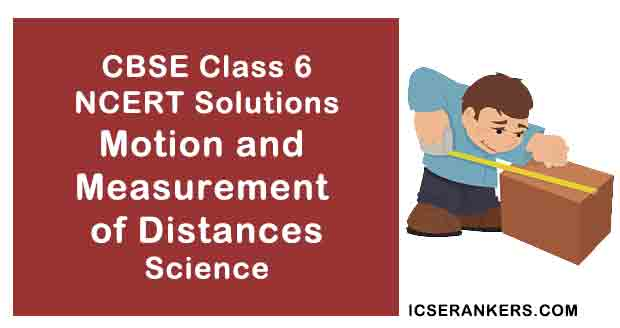# NCERT Solutions for Chapter 10 Motion and Measurement of Distances Class 6 Science

Short Notes for Class 6 Science Chapter 10 Motion and Measurement of Distances

Different modes of transport are used to go from one place to another. In ancient times, people used length of a foot, the width of a finger, the distance of a step as units of measurement. This caused confusion and a need to develop a uniform system of measurement arose. Now, we use International System of Units (SI units). This is accepted all over the world. Metre is the unit of length in SI unit Motion in a straight line is called rectilinear motion. In circular motion an object moves such that its distance from a fixed point remains the same. Motion that repeats itself after some period of time is called periodic motion.Motion and Measurement of Distances Questions and Answers

 Chapter Name Motion and Measurement of Distances NCERT Solutions Class CBSE Class 6 Textbook Name Science Related Readings NCERT Solutions for Class 6NCERT Solutions for Class 6 ScienceSummary of Motion and Measurement of Distances

Question 1: Give two examples each, of modes of transport used on land, water and air.

Land transport: Bus, train, motorbike.

Water transport: Boat, ship, steamer.

Air Transport: Aeroplane, Helicopter.

Question 2: Fill in the blanks:

(i) One metre is ________ cm.

(ii) Five kilometre is ________ m.

(iii)Motion of a child on a swing is _________.

(iv)Motion of the needle of a sewing machine is _________.

(v) Motion of wheel of a bicycle is__________.

(i) One metre is 100 cm.

(ii) Five kilometre is 5000 m.

(iii)Motion of a child on a swing is periodic motion.

(iv)Motion of the needle of a sewing machine is periodic motion.

(v) Motion of wheel of a bicycle is circular motion.

Question 3: Why can a pace or a footstep not be used as a standard unit of length?

A pace or a footstep not be used as a standard unit of length because the size of pace and footstep vary from person to person. This will lead to confusion while measuring the lengths by different persons. We should use standard units like International System of Units (SI Units).

Question 4: Arrange the following lengths in their increasing magnitude:

1 metre, 1 centimetre, 1 kilometre, 1 millimetre.

1 millimetre, 1 centimetre, 1 metre, 1 kilometre.

Question 5: The height of a person is 1.65 m. Express it into cm and mm.

We know that 1 metre = 100 cm and 1 metre = 1000 mm

So, 1.65 m = 1.65 × 100 = 165 cm

and 1.65 m = 1.65 × 1000 = 1650 mm

Question 6: The distance between Radha's home and her school is 3250 m. Express this distance into km.

We know that 1 m = 1/1000 km

So, 3250 m = 3250/1000 km = 3.25 km

Question 7: While measuring the length of a knitting needle, the reading of the scale at one end is 3.0 cm and at the other end is 33.1 cm. What is the length of the needle?

The length of the needle = 33.1 cm – 3.0 cm = 30.1 cm

Question 8: Write the similarities and differences between the motion of a bicycle and a ceiling fan that has been switched on.

Similarity: Both show a circular motion.

Difference: Bicycle shows a rectilinear motion whereas a ceiling fan does not.

Question 9: Why could you not use an elastic measuring tape to measure distance? What would be some of the problems you would meet in telling someone about a distance you measured with an elastic tape?